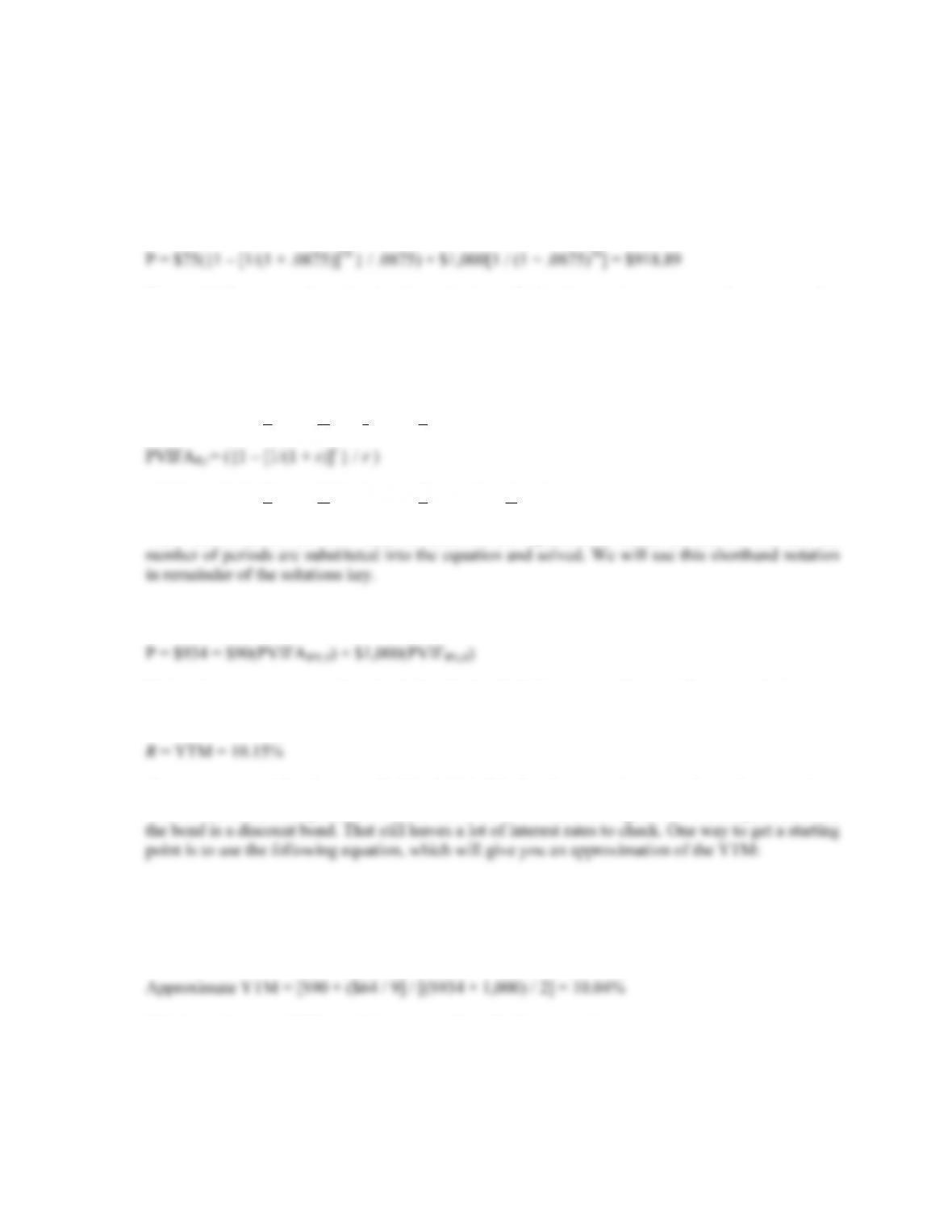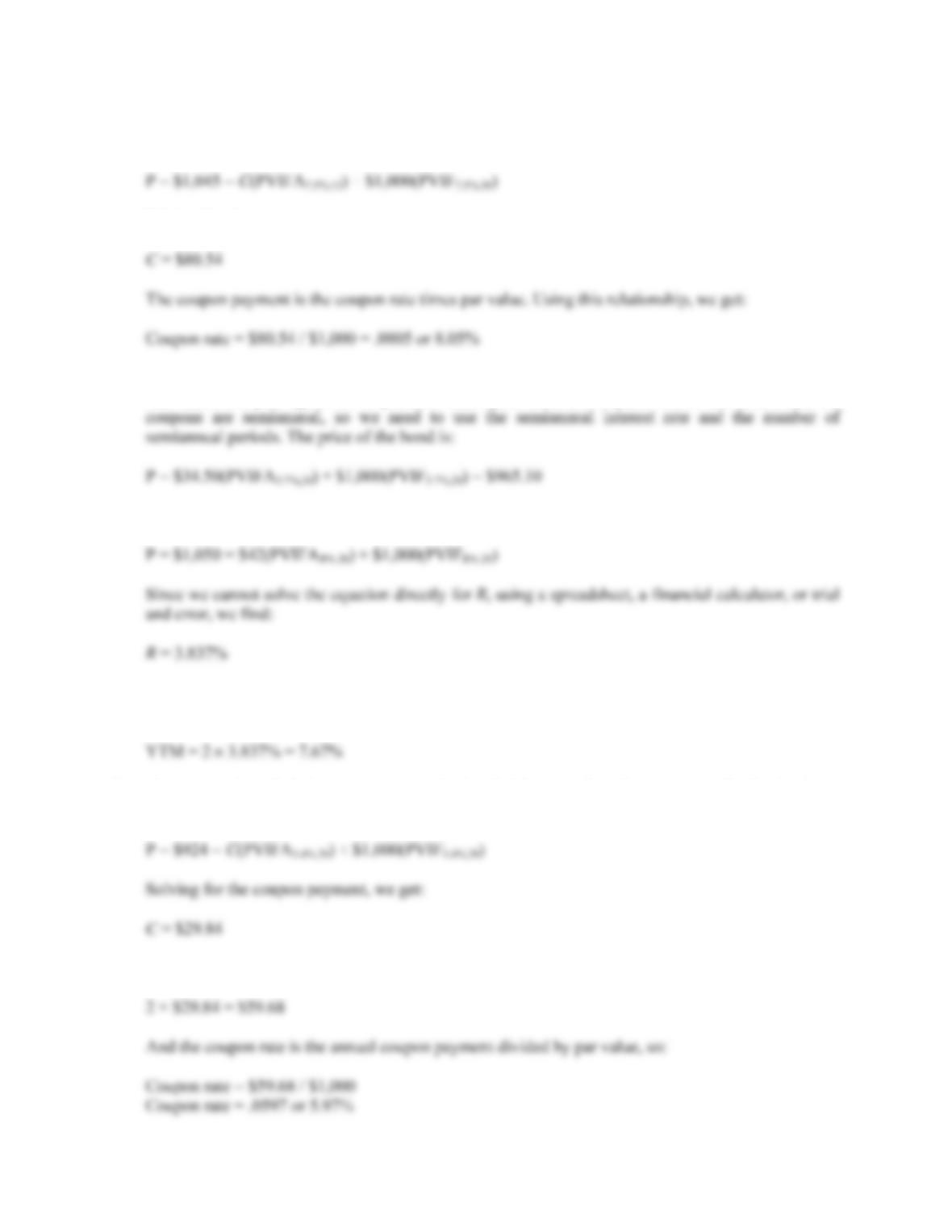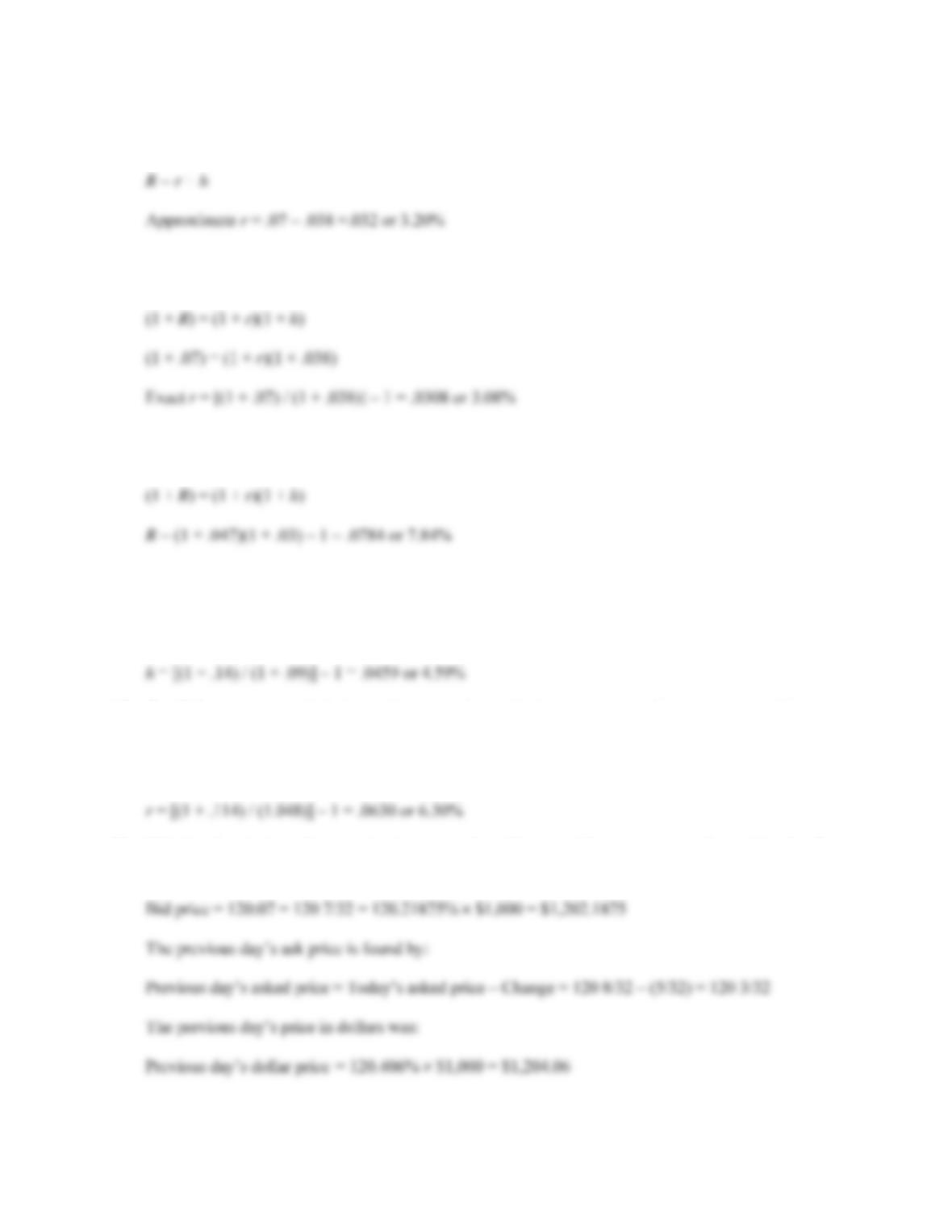Type
Quiz
Book Title
Fundamentals of Corporate Finance Standard Edition 9th Edition
ISBN 13
978-0073382395

### 978-0073382395 Chapter 7 Questions and Problems 1-13

April 3, 2019CHAPTER 7 B-119
10. The term structure is based on pure discount bonds. The yield curve is based on coupon-bearing
issues.
11. Bond ratings have a subjective factor to them. Split ratings reflect a difference of opinion among
credit agencies.
12. As a general constitutional principle, the federal government cannot tax the states without their
consent if doing so would interfere with state government functions. At one time, this principle was
thought to provide for the tax-exempt status of municipal interest payments. However, modern court
rulings make it clear that Congress can revoke the municipal exemption, so the only basis now
appears to be historical precedent. The fact that the states and the federal government do not tax each
other’s securities is referred to as “reciprocal immunity.”
13. Lack of transparency means that a buyer or seller can’t see recent transactions, so it is much harder
to determine what the best bid and ask prices are at any point in time.
14. Companies charge that bond rating agencies are pressuring them to pay for bond ratings. When a
company pays for a rating, it has the opportunity to make its case for a particular rating. With an
unsolicited rating, the company has no input.
15. A 100-year bond looks like a share of preferred stock. In particular, it is a loan with a life that almost
certainly exceeds the life of the lender, assuming that the lender is an individual. With a junk bond,
the credit risk can be so high that the borrower is almost certain to default, meaning that the creditors
are very likely to end up as part owners of the business. In both cases, the “equity in disguise” has a
Solutions to Questions and Problems
NOTE: All end of chapter problems were solved using a spreadsheet. Many problems require multiple
steps. Due to space and readability constraints, when these intermediate steps are included in this
solutions manual, rounding may appear to have occurred. However, the final answer for each problem is
found without rounding during any step in the problem.
Basic
1. The yield to maturity is the required rate of return on a bond expressed as a nominal annual interest
rate. For noncallable bonds, the yield to maturity and required rate of return are interchangeable
terms. Unlike YTM and required return, the coupon rate is not a return used as the interest rate in
2. Price and yield move in opposite directions; if interest rates rise, the price of the bond will fall. This
is because the fixed coupon payments determined by the fixed coupon rate are not as valuable when
interest rates rise—hence, the price of the bond decreases.B-120 SOLUTIONS
NOTE: Most problems do not explicitly list a par value for bonds. Even though a bond can have any par
value, in general, corporate bonds in the United States will have a par value of \$1,000. We will use this
par value in all problems unless a different par value is explicitly stated.
3. The price of any bond is the PV of the interest payment, plus the PV of the par value. Notice this
problem assumes an annual coupon. The price of the bond will be:
We would like to introduce shorthand notation here. Rather than write (or type, as the case may be)
the entire equation for the PV of a lump sum, or the PVA equation, it is common to abbreviate the
equations as:
PVIFR,t = 1 / (1 + r)t
which stands for Present Value Interest Factor
which stands for Present Value Interest Factor of an Annuity
These abbreviations are short hand notation for the equations in which the interest rate and the
4. Here we need to find the YTM of a bond. The equation for the bond price is:
Notice the equation cannot be solved directly for R. Using a spreadsheet, a financial calculator, or
trial and error, we find:
If you are using trial and error to find the YTM of the bond, you might be wondering how to pick an
interest rate to start the process. First, we know the YTM has to be higher than the coupon rate since
Approximate YTM = [Annual interest payment + (Price difference from par / Years to maturity)] /
[(Price + Par value) / 2]
Solving for this problem, we get:
This is not the exact YTM, but it is close, and it will give you a place to start.CHAPTER 7 B-121
5. Here we need to find the coupon rate of the bond. All we need to do is to set up the bond pricing
equation and solve for the coupon payment as follows:
Solving for the coupon payment, we get:
6. To find the price of this bond, we need to realize that the maturity of the bond is 10 years. The bond
was issued one year ago, with 11 years to maturity, so there are 10 years left on the bond. Also, the
7. Here we are finding the YTM of a semiannual coupon bond. The bond price equation is:
Since the coupon payments are semiannual, this is the semiannual interest rate. The YTM is the APR
of the bond, so:
8. Here we need to find the coupon rate of the bond. All we need to do is to set up the bond pricing
equation and solve for the coupon payment as follows:
Since this is the semiannual payment, the annual coupon payment is:B-122 SOLUTIONS
9. The approximate relationship between nominal interest rates (R), real interest rates (r), and inflation
(h) is:
The Fisher equation, which shows the exact relationship between nominal interest rates, real interest
rates, and inflation is:
10. The Fisher equation, which shows the exact relationship between nominal interest rates, real interest
rates, and inflation is:
11. The Fisher equation, which shows the exact relationship between nominal interest rates, real interest
rates, and inflation is:
(1 + R) = (1 + r)(1 + h)
12. The Fisher equation, which shows the exact relationship between nominal interest rates, real interest
rates, and inflation is:
(1 + R) = (1 + r)(1 + h)
13. This is a bond since the maturity is greater than 10 years. The coupon rate, located in the first
column of the quote is 6.125%. The bid price is: